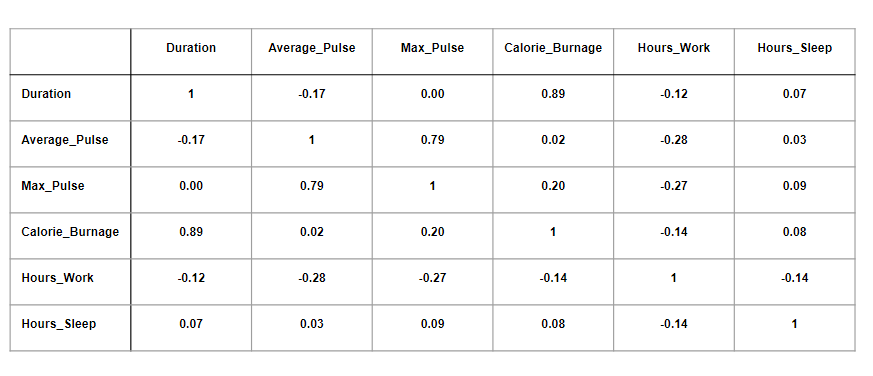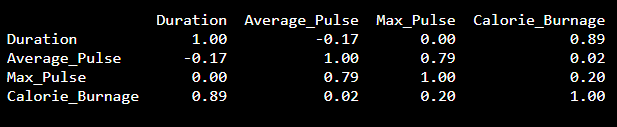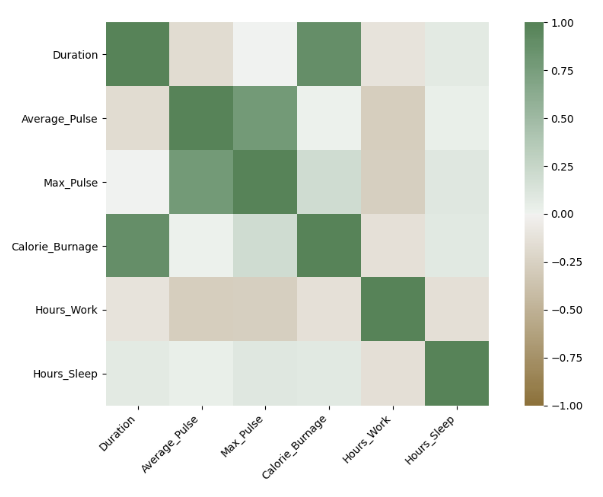# Data Science - Statistics Correlation Matrix

## Correlation Matrix

A matrix is an array of numbers arranged in rows and columns.

A correlation matrix is simply a table showing the correlation coefficients between variables.

Here, the variables are represented in the first row, and in the first column:The table above has used data from the full health data set.

Observations:

• We observe that Duration and Calorie_Burnage are closely related, with a correlation coefficient of 0.89. This makes sense as the longer we train, the more calories we burn
• We observe that there is almost no linear relationships between Average_Pulse and Calorie_Burnage (correlation coefficient of 0.02)
• Can we conclude that Average_Pulse does not affect Calorie_Burnage? No. We will come back to answer this question later!

## Correlation Matrix in Python

We can use the `corr()` function in Python to create a correlation matrix. We also use the `round()` function to round the output to two decimals:

### Example

Corr_Matrix = round(full_health_data.corr(),2)
print(Corr_Matrix)
Try it Yourself »

Output:## Using a Heatmap

We can use a Heatmap to Visualize the Correlation Between Variables:The closer the correlation coefficient is to 1, the greener the squares get.

The closer the correlation coefficient is to -1, the browner the squares get.

## Use Seaborn to Create a Heatmap

We can use the Seaborn library to create a correlation heat map (Seaborn is a visualization library based on matplotlib):

### Example

import matplotlib.pyplot as plt
import seaborn as sns

correlation_full_health = full_health_data.corr()

axis_corr = sns.heatmap(
correlation_full_health,
vmin=-1, vmax=1, center=0,
cmap=sns.diverging_palette(50, 500, n=500),
square=True
)

plt.show()
Try it Yourself »

### Example Explained:

• Import the library seaborn as sns.
• Use the full_health_data set.
• Use sns.heatmap() to tell Python that we want a heatmap to visualize the correlation matrix.
• Use the correlation matrix. Define the maximal and minimal values of the heatmap. Define that 0 is the center.
• Define the colors with sns.diverging_palette. n=500 means that we want 500 types of color in the same color palette.
• square = True means that we want to see squares.